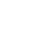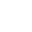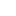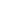# Kruskal’s Algorithm – Minimum Spanning Tree (MST) – Complete Java Implementation

What is Kruskal Algorithm?

• Kruskal’s algorithm for finding the Minimum Spanning Tree(MST), which finds an edge of the least possible weight that connects any two trees in the forest
• It is a greedy algorithm.
• It finds a subset of the edges that forms a tree that includes every vertex, where the total weight of all the edges in the tree is minimized.
• If the graph is not connected, then it finds a minimum spanning forest (a minimum spanning tree for each connected component).
• Number of edges in MST: V-1 (V – no of vertices in Graph).

Example:How this algorithm works?

We strongly recommend reading Find Cycle in Undirected Graph using Disjoint Set (Union-Find) before continue.

1. Sort the edges in ascending order of weights.
2. Pick the edge with the least weight. Check if including this edge in spanning tree will form a cycle is Yes then ignore it if No then add it to spanning tree.
3. Repeat the step 2 till spanning tree has V-1 (V – no of vertices in Graph).
4. Spanning tree with least weight will be formed, called Minimum Spanning Tree

Pseudo Code:

```KRUSKAL(G):
A = ∅
foreach v ∈ G.V:
MAKE-SET(v)
foreach (u, v) in G.E ordered by weight(u, v), increasing:
if FIND-SET(u) ≠ FIND-SET(v):
A = A ∪ {(u, v)}
UNION(u, v)
return A
```

See the animation below for more understanding.JavaCode:

Output:

```Minimum Spanning Tree:
Edge-0 source: 1 destination: 2 weight: 1
Edge-1 source: 1 destination: 3 weight: 2
Edge-2 source: 3 destination: 4 weight: 2
Edge-3 source: 0 destination: 2 weight: 3
Edge-4 source: 4 destination: 5 weight: 6
```